# Comparison of Test Data and CFD

In this section comparisons of the CFD results and test data are organized as follows:

• Comparisons of two different approaches for modeling the turbulence/combustion interaction, namely: steady flamelet model and simplified Conditional Moment Closure (designated as CMC -model in the figures from here on) obtained with the classical Smagorinsky subgrid scale model,
• Comparisons of two subgrid-scale models, namely: classical Smagorinsky subgrid scale model and dynamic Smagorinsky one using the steady flamelet model of turbulence/combustion interaction.

Fig.6. shows mean velocity axial component and mixture fraction along the centerline for both steady flamelet and CMC approaches. One can see quite significant discrepancies between both models. Steady flamelet shows rapid velocity decay in the near field and then the slope is quite close to the one measured experimentally. On the other hand the CMC model leads to much smaller velocity decay. The velocity profile for CMC is closer to experimental data but the slope at the distance z/D=10 is underpredicted. At the distance z/D=30 both models predict good velocity decay. As to the mixture fraction both models predict quite a long distance z/D ≤ 16 for which the mixture fraction is unity while the experiment showed much more intense mixing in this region. As a consequence, at the jet centerline for a distance z/D ≤ 16 the numerical models do not predict reaction and this is reflected in the temperature profile and combustion products like CO2 shown in Fig.8. However, further downstream in the fully developed flame the numerical results are much closer to the experimental data. Especially CMC predicts the value of temperature maximum and its location with quite high accuracy (see Fig.7).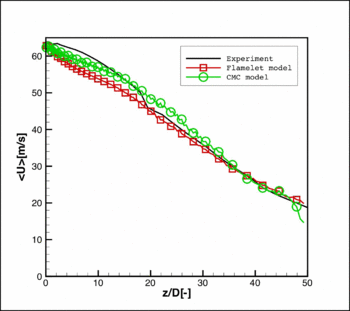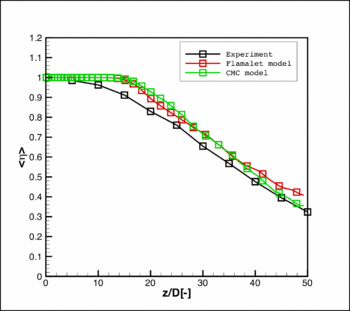Fig. 6. Axial velocity (left) and mixture fraction (right) along the flame axis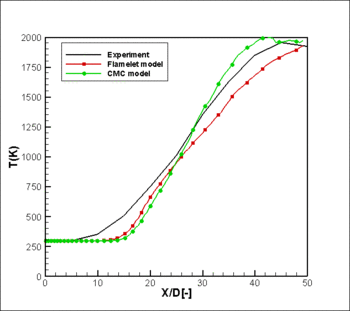Fig. 7. Temperature along the flame axis

The species distributions are shown in Figs 8-10. As the models predict the flame too far from the nozzle exit, in all species distributions similar discrepancies are observed at the distance z/D = 10 – 20. And again, as was observed for the temperature profile, further in the developed flame the agreement with experimental data is much better. The distributions for H2O, O2, CO predicted with CMC are nearly perfect. A bit worse results were obtained for H2.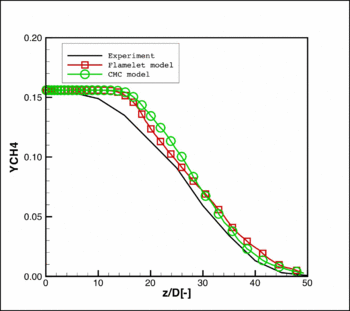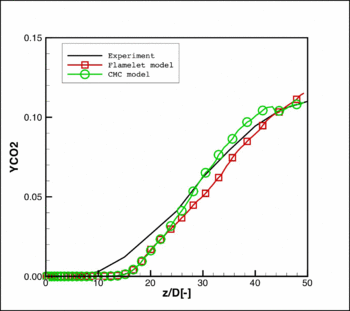Fig. 8. Mean mass fraction of CH4 (left) and CO2 (right)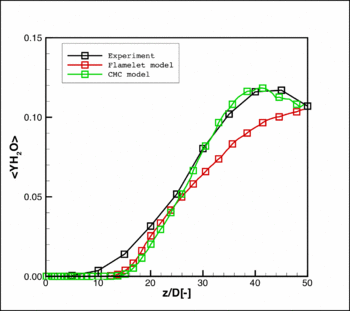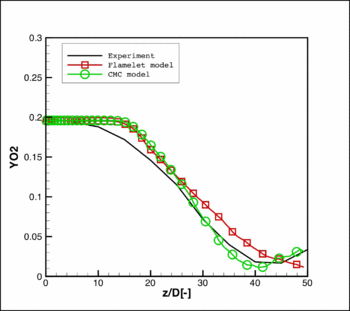Fig. 9. Mean mass fraction of H2O (left) and O2 (right)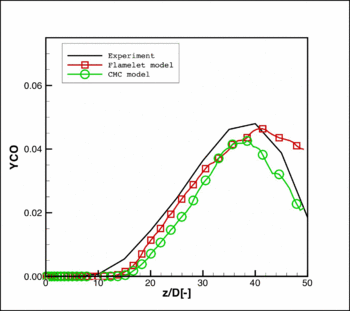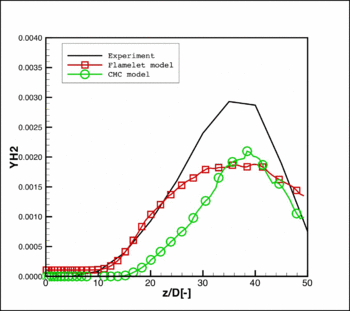Fig. 10. Mean mass fraction of CO (left) and H2 (right)

Fig.11 shows the fluctuating axial velocity and mixture fraction components. In the near field the velocity fluctuations predicted by both models at the distance z/D ≤ 10 are much higher than measured experimentally which is consistent with much faster velocity decay in this region shown in Fig.6. Further downstream in the developed flame, agreement is very good. For the fluctuating component of the mixture fraction shown in Fig.11 in the near field fluctuations are lower than observed experimentally and again in the developed flame agreement is quite good. Maximum value of temperature fluctuations (Fig.12) is located much closer to the nozzle exit than the maximum value of mean temperature. CMC leads to higher level of temperature fluctuations. Fluctuating components of major species are shown in Figs 13-15. Both models predict reasonable levels of species fluctuation in agreement with experimental results.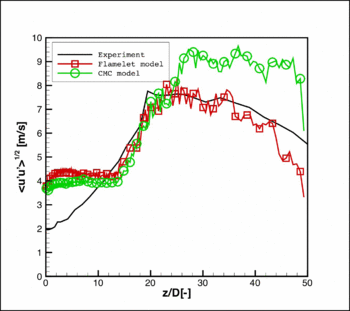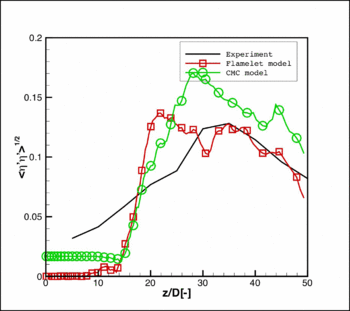Fig. 11. RMS of axial velocity (left) and mixture fraction (right) along the jet flame axis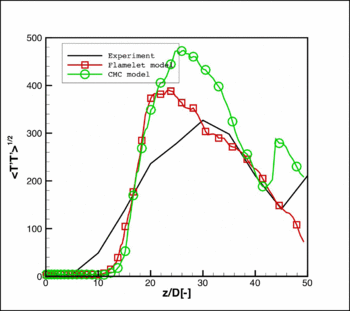Fig. 12. RMS of temperature along the jet flame axis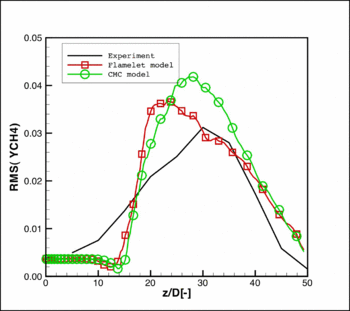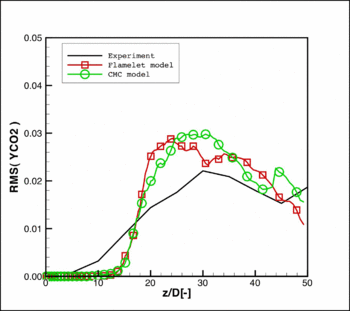Fig. 13. RMS of mass fraction of CH4 (left) and CO2 (right)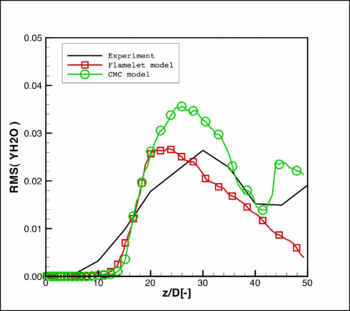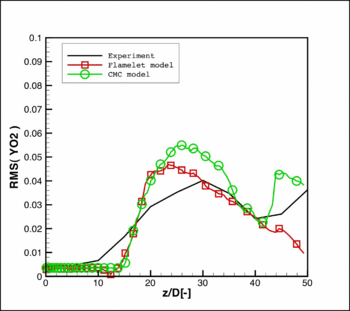Fig. 14. RMS of mass fraction of H2O (left) and O2 (right)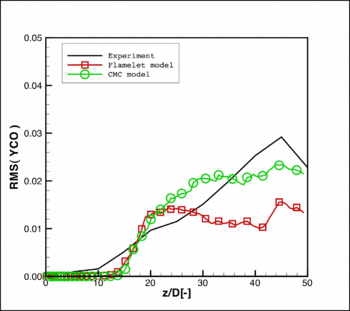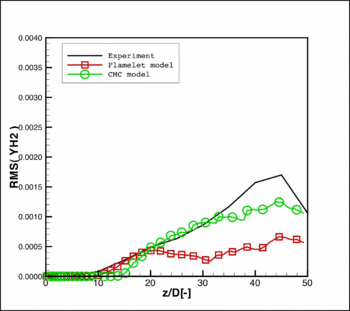Fig. 15. RMS of mass fraction of CO (left) and H2 (right)

Sample radial profiles of mean and fluctuating velocities are shown in Figs 16 and 17. Both models predict radial velocity profiles in very good agreement with experiment in all the cross sections. Fluctuating components are also in good agreement with experimental data. At the last cross section at the distance z/D = 45 the flamelet model leads to some overprediction of fluctuating components far from the flame axis. Mean mixture fraction shown in Fig.18 is close to experimental data. As to the mixture fraction fluctuating component shown in Fig. 19 agreement with experimental data is also reasonable. However, a surprising result was obtained at the distance z/D = 30 where the radial distribution of fluctuating component of mixture fraction obtained with the CMC is qualitatively different from experimental profile and from the result obtained with steady flamelet. In the profile predicted with CMC a local maximum is observed on the axis that is not present in experimental data. At the current stage of the research it seems to be difficult to explain these discrepancies analyzing physics of turbulence/combustion interaction.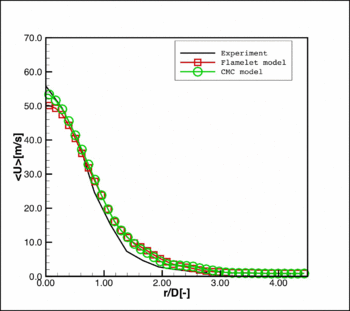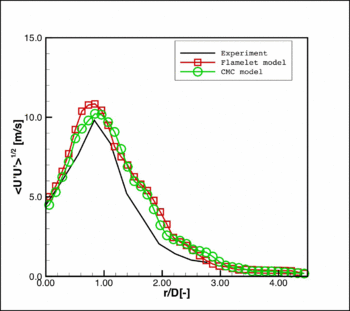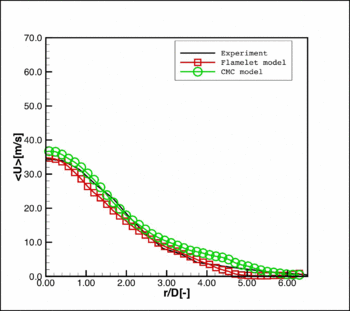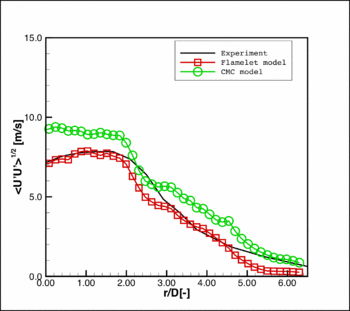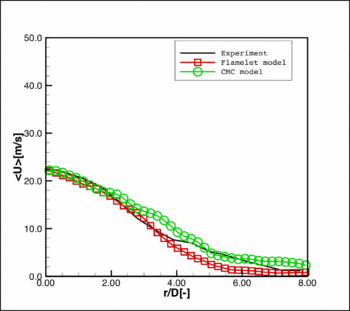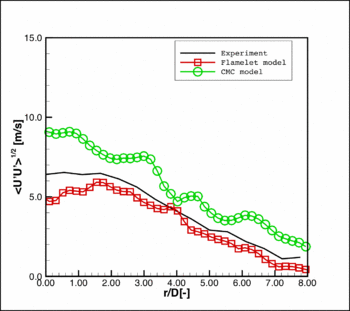Fig 16. Mean axial velocity radial profile at the cross sections (a) z/D=15, (b) z/D=30, (c) z/D=45 Fig 17. Fluctuating axial velocity radial profile at the cross sections (a) z/D=15, (b) z/D=30, (c) z/D=45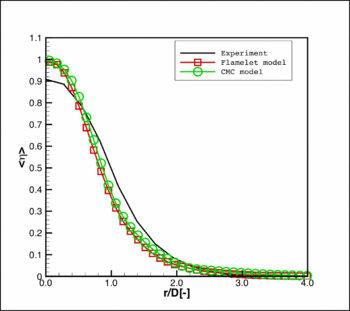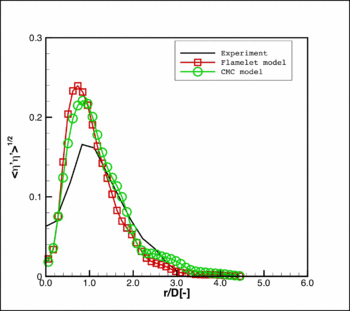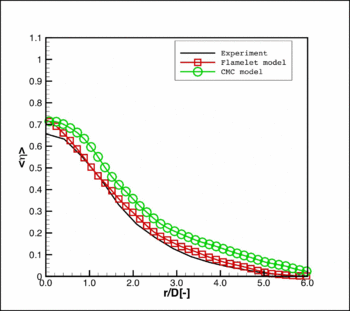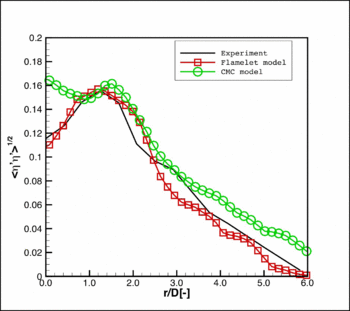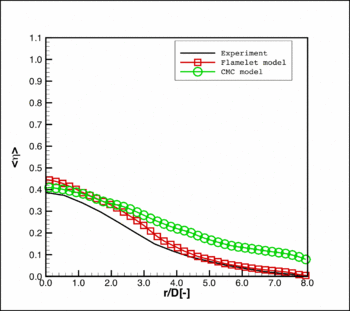Fig 18. Mean mixture fraction radial profile at the cross sections (a) z/D=15, (b) z/D=30, (c) z/D=45 Fig 19. Fluctuating mixture fraction radial profile at the cross sections (a) z/D=15, (b) z/D=30, (c) z/D=45

LES calculations were also performed to evaluate the importance of the SGS model with Smagorinsky and dynamic Smagorinsky models using in both cases the steady flamelet approach. The mean profile of axial velocity and mean mixture fraction along the flame axis are shown in Fig. 20. Both SGS models predict very similar velocity profiles especially in the near field where only mixing appears without combustion. More clear differences are observed in the region of combustion z/D > 20. A surprising conclusion is that the simple Smagorinsky model predicts better the mean velocity profile than the dynamic one. The mean mixture fraction profile obtained with the Smagorisnky model is also closer to the experimental results than the profile obtained with the dynamic model. The same conclusion that Germano model leads to worse results can be derived from the analysis of the temperature profile shown in Fig. 21.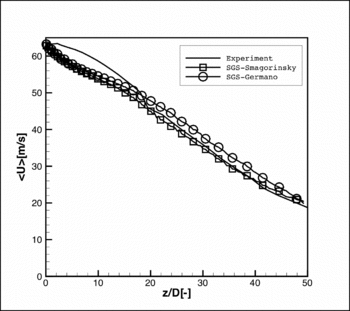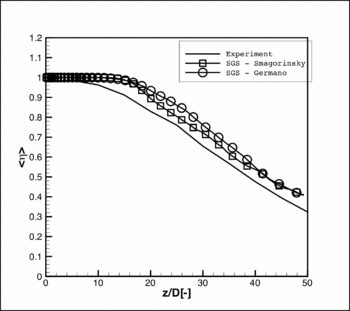Fig. 20. Axial velocity (left) and mixture fraction (right) along the flame axis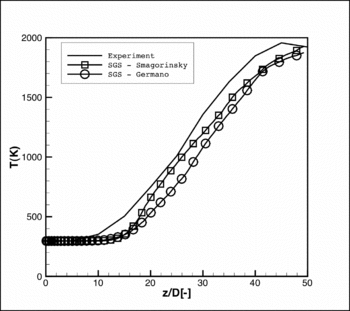Fig. 21. Temperature along the flame axis

Figs 22-24 show mean mass fraction of major species. In all cases agreement with experimental data is satisfactory, and in all cases results are slightly better for the Smagorinsky SGS model.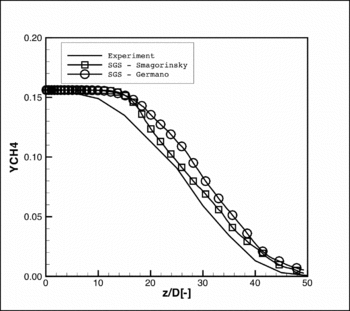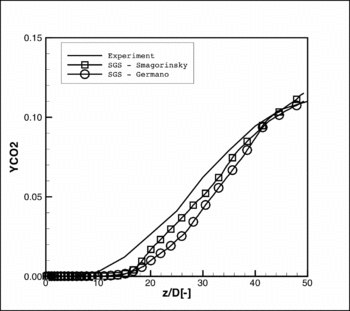Fig. 22. Mean mass fraction of CH4 (left) and CO2 (right)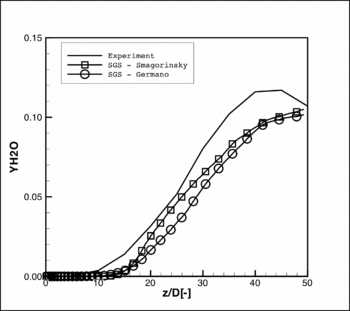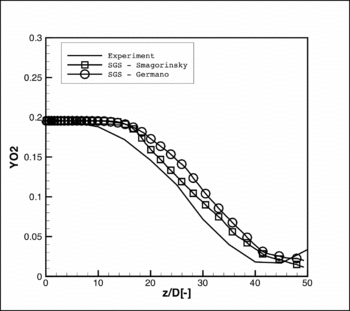Fig. 23. Mean mass fraction of H2O (left) and O2 (right)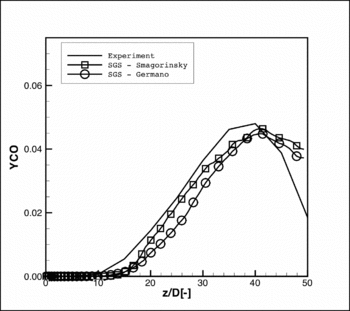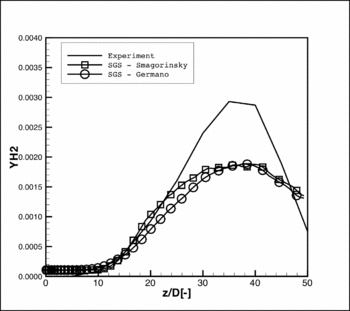Fig. 24. Mean mass fraction of CO (left) and H2 (right)

Fluctuating components of the axial velocity and mixture fraction are illustrated in Fig. 25 & 26. Smagorinsky subgrid-scale model leads also to better prediction of the fluctuating component of axial velocity especially in the flame region. For mixture fraction fluctuations the SGS model does not introduce significant differences. As to the temperature fluctuations, the influence of the SGS model also is not very important.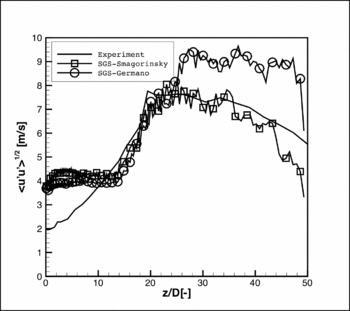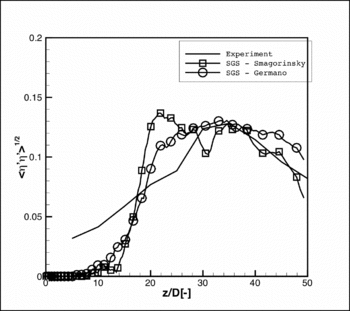Fig. 25. RMS of axial velocity (left) and mixture fraction (right) along the jet flame axis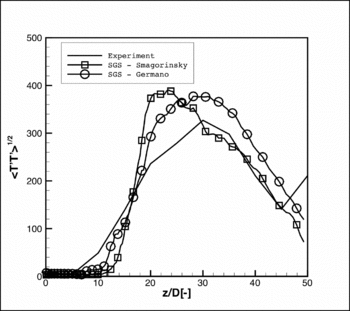Fig. 26. RMS of temperature along the jet flame axis

The maximum value of the fluctuating component is predicted upstream with the use of the Smagorinsky model compared to the dynamic one. Fluctuations of the major species shown in Figs 27 – 29 are similarly obtained with both SGS models.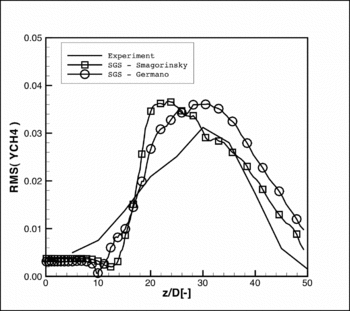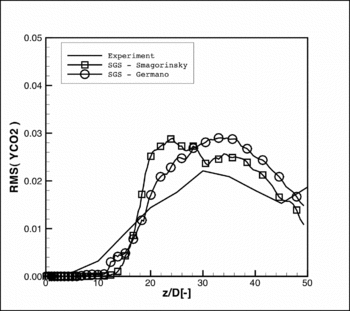Fig. 27. RMS of mass fraction of CH4 (left) and CO2 (right)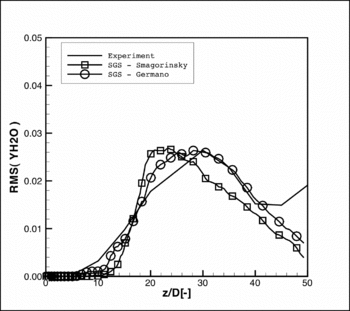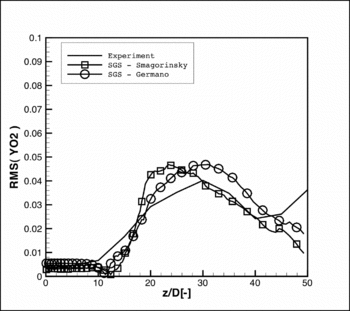Fig. 28. RMS of mass fraction of H2O (left) and O2 (right)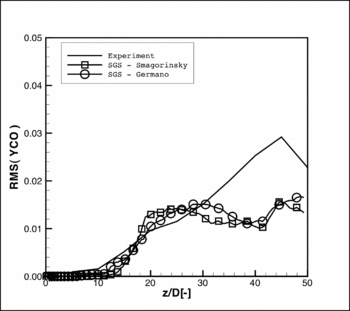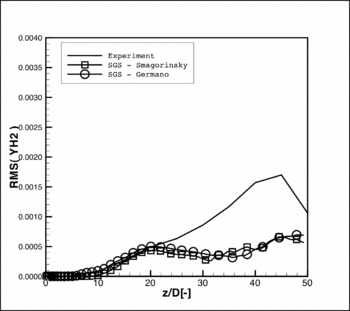Fig. 29. RMS of mass fraction of CO (left) and H2 (right)

Contributed by: Andrzej Boguslawski, Artur Tyliszczak — Częstochowa University of Technology